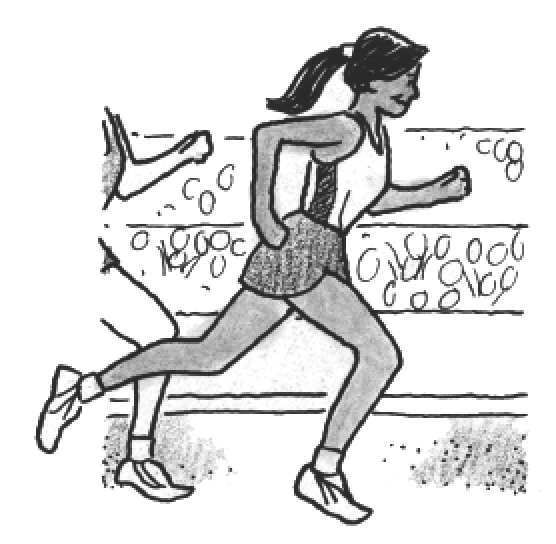Home > APCALC > Chapter 1 > Lesson 1.2.3 > Problem1-55

1-55.

A marathon runner runs a $26.2$-mile race. Her distance traveled in miles after $t$ hours is $p(t) = 7t$

1. How long does it take her to finish the race?

$26.2 = p(t)$
Solve for $t$.

2. What is her average velocity? Explain your reasoning.

$\text{average velocity }= \frac{\Delta \text{ distance}}{\Delta \text{time}}$

Evaluate and explain.

3. Suppose she runs at a constant pace of $7$ miles/hour. How far will she have gone in $2$ hours?

$\text{velocity }= \frac{\text{ distance}}{ \text{time}}$

Evaluate.

4. Show how the units in your answer to part (c) reduce using $\text{(rate)(time)} = \text{distance}$.

$\text{(rate)(time)}= \text{(miles/hour)(hours)} =$ _________